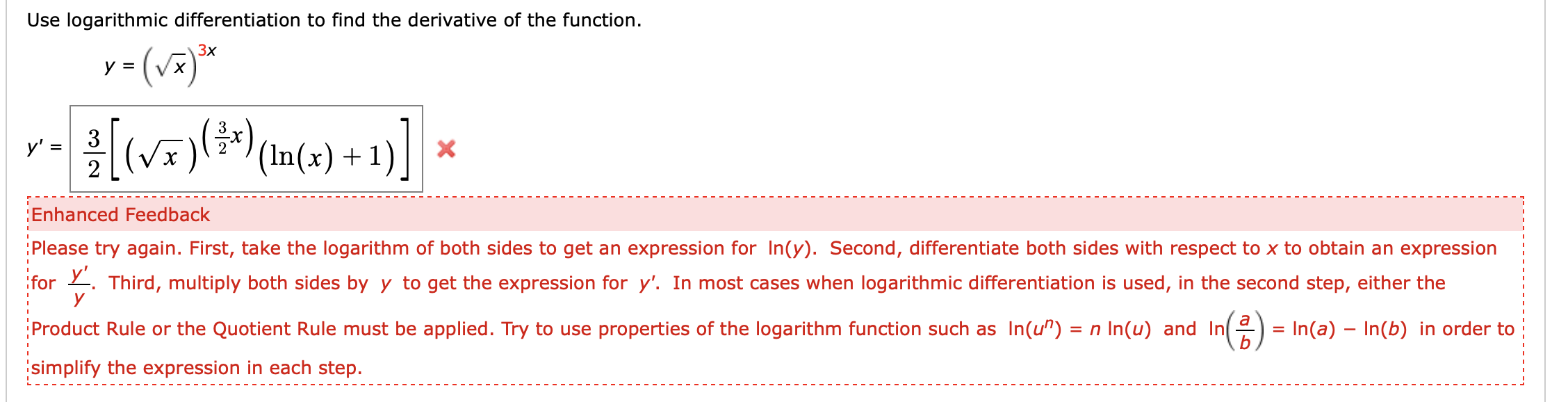# Use logarithmic differentiation to find the derivative of the functiony (vЗxVx3(V)у' -2X'(In(x) 1Enhanced FeedbackPlease try again. First, take the logarithm of both sides to get an expression for In(y). Second, differentiate both sides with respect to x to obtain an expressionfor Third, multiply both sides byy to get the expression for y'. In most cases when logarithmic differentiation is used, in the second step, either theуProduct Rule or the Quotient Rule must be applied. Try to use properties of the logarithm function such as In(u")n In(u) and In= In(a) In(b) in order tosimplify the expression in each step.

Question
65 viewshelp_outlineImage TranscriptioncloseUse logarithmic differentiation to find the derivative of the function y (v Зx Vx 3 (V) у' - 2 X '(In(x) 1 Enhanced Feedback Please try again. First, take the logarithm of both sides to get an expression for In(y). Second, differentiate both sides with respect to x to obtain an expression for Third, multiply both sides byy to get the expression for y'. In most cases when logarithmic differentiation is used, in the second step, either the у Product Rule or the Quotient Rule must be applied. Try to use properties of the logarithm function such as In(u") n In(u) and In = In(a) In(b) in order to simplify the expression in each step. fullscreen
check_circle

Step 1

To differentiate the following function

Step 2

Taking logarithm both side

Step 3

Differentiate both sides with respect to...

### Want to see the full answer?

See Solution

#### Want to see this answer and more?

Solutions are written by subject experts who are available 24/7. Questions are typically answered within 1 hour.*

See Solution
*Response times may vary by subject and question.
Tagged in